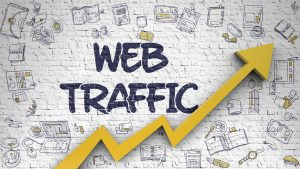### What is Time Series Analysis?

‘Time’ is the most important factor which ensures success in a business. It’s difficult to keep up with the pace of time.  But, technology has developed some powerful methods using which we can ‘see things’ ahead of time!

Nope, not the time machine, we are talking about the methods of prediction & forecasting. As the name ‘time series forecasting’ suggests, it involves working on time (years, days, hours, minutes) based data, to derive hidden insights to make informed decision making.

### Key Takeaways from Time Series Forecasting using Python Course?

#### This course is designed for people who want to solve problems related to Time Series Forecasting. By the end of the course, you will learn to apply the following necessary skills and techniques required to solve Time Series problems:

• Machine Learning for Time Series forecasting

• Exponential Smoothing Methods

• Framework to evaluate Time Series Models

• ARIMA and SARIMA Model

• Tuning Parameters for ARIMA

• Deep Learning for time series

### Projects

Forecasting the daily count of passengers using JetRailUsing Time series models for forecasting energy consumptionForecasting web Traffic using Deep LearningUsing Time series models for Sales Forecasting### Course curriculum

• 1
##### Module 1: Overview of the Course
• Overview of the course
• Instructor Introduction
• Getting to know you
• Handouts
• 2
##### Module 2: Introduction to TIme Series
• What is Time Series?
• Quiz: What is Time Series?
• Application of Time Series
• Quiz: Applications of Time Series
• Univariate and Multivariate Time Series
• Quiz: Univariate and Multivariate Time Series
• Time Series with Features
• Quiz: Time Series with Features
• 3
##### Module 3: Working with Time Series
• Working with Time Series using pandas
• Quiz: Working with Time Series using pandas
• Reading and Plotting time series
• Reasmpling in Time Series
• Quiz: Reasmpling in Time Series
• Dealing with different Time Zones in time series
• 4
##### Module 4: Build your first time series model
• What is Time Series Forecasting?
• Quiz: Time Series Forecasting
• Defining the Problem Statement
• Evaluation Metrics
• Quiz: Evaluation Metrics
• Validation techniques
• Quiz: Validation techniques
• Feature Extraction for time series
• Quiz: Feature extraction
• Linear regression model on time series
• Quiz: Linear Regression for time series
• 5
##### Module 5: Simple time series forecasting models
• Naive Model
• Quiz: Naive Model
• Simple Average
• Quiz: Simple Average
• Moving Average
• Quiz: Moving Average
• Weighted Moving Average
• Quiz: Weighted Moving Average
• 6
##### Module 6: Exponential Smoothing Models
• Simple Exponential Smoothing
• Quiz: Simple Exponential Smoothing
• Time Series Components
• Time Series Components
• Double Exponential Smoothing
• Quiz: Double Exponential Smoothing
• Holt Winters (TES)
• Quiz: Holt Winters (TES)
• 7
##### Assignment
• Forecasting Energy Consumtion
• 8
##### Module 7: Arima model and Stationarity for Time Series
• Inrodcution to ARIMA
• Quiz: Inrodcution to ARIMA
• Stationarity in Time Series
• Quiz: Stationarity in Time Series
• Tests for Stationarity
• Quiz: Tests for Stationarity
• Methods to make series stationary
• Quiz: Methods to make series stationary
• Parameters of ARIMA
• Quiz: Parameters of ARIMA
• AR and MA models
• ARIMA
• Quiz: ARIMA
• SARIMA
• Quiz: SARIMA
• 9
##### Assignment
• Forecasting Energy Consumption
• 10
##### Module 8: Prophet
• Seasonal Forecasting with Prophet
• Quiz: Seasonal Forecasting with Prophet
• 11
##### Module 9: Project - Sales Forecasting
• Understanding Problem Statement
• Data Preprocessing
• Feature Extraction and Data Exploration
• Building Time Series Forecasting Model
• Building Machine Learning Models
• Forecasting Sales
• 12
##### What's Next
• What's Next
• What's Next in the Program?

### What do I need to take Time Series Forecasting course?

• A working laptop / desktop with 4 GB RAM
• A working Internet connection
• Basic knowledge of Machine Learning
• Basic knowledge of Python - check out this Course### FAQ

• Who should take this course?

This course is meant for people looking to explore Time Series Forecasting in Python.

• Do I need to install any software before starting the course?

You will need to download and install python.

• Do I need to take the modules in a specific order?

We would highly recommend taking the course in the order in which it has been designed to gain the maximum knowledge from it.